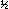Scheduling with Batching: Two Job Types

## Abstract

In this paper we present an algorithm for the 2-weight batching problem: given njobs with weight w, and njobs with weight w, all having processing time p, and given a batch setup time, find a sequence of batches of jobs such that the weighted sum of the n = n+ njob completion times is minimized. The algorithm has running time O(nlogn) when p andare fixed; previously, the fastest known algorithm for this problem had running time O(n). Various properties of optimal solutions are exploited to reduce the problem to one of finding the minimum entry in a certain matrix. Since this matrix has some strong structural properties, its minimum entry can be found in time that is a sublinear function of the matrix size.